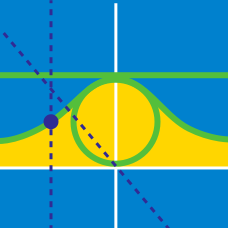Calculus

# Parametric Equations - Area

What is the area between the $x$-axis and the parametric curve $x=6\cos \theta, y=7\sin \theta$ in the interval $0 \leq \theta \leq \pi?$

What is the area of the region bounded by the $x$-axis and the parametric curve $\begin{array}{c}& x=t^2 & y=3+18t-5t^2 \end{array}$ in the interval $0 \le t \le 4?$

What is the area between the $x$-axis and the parametric curve defined by $x=t, y=-t^2+11?$

What is the area between the $x$-axis and the parametric curve defined by $x=5t^3$ and $y=e^t$ in the interval $0 \leq t \leq 3?$

Let $a, b, k$ be positive integers such that $0 < a < b < k$. Consider the points $P=(-a, ka)$ and $Q=(b, kb)$ which lie on the graph of $y=k\lvert x \rvert$. If the area of the region bounded by $y=k\lvert x \rvert$ and the line segment $PQ$ is $95$, what is $k$?

×